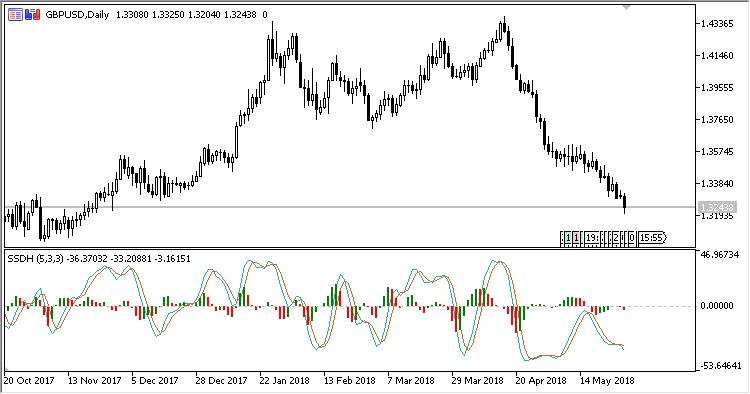# SSD_With_Histogram – indicator for MetaTrader 5

The indicator displays in a separate window the slow stochastic line, a signal line plotted by the slow line smoothing method, and a histogram showing the difference between the slow line and the signal line.

It has five input parameters:

• %K period – stochastic K line calculation period;
• %D period – stochastic D line calculation period;
• Slowing – slowing period;
• Method – stochastic calculation method;
• Price field – stochastic calculation price.
Related Posts

Calculations:

```K = Stochastic D - 50.0
D = SMA(K, %D Period)
Histogram = K - D
```